# Unitary space

(diff) ← Older revision | Latest revision (diff) | Newer revision → (diff)

A vector space over the fieldof complex numbers, on which there is given an inner product of vectors (where the product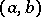of two vectorsandis, in general, a complex number) that satisfies the following axioms:

1)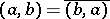;

2);

3)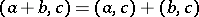;

4) if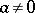, then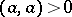, i.e. the scalar square of a non-zero vector is a positive real number.

A unitary space need not be finite-dimensional. In a unitary space one can, just as in Euclidean spaces, introduce the concept of orthogonality and of an orthonormal system of vectors, and in the finite-dimensional case one can prove the existence of an orthonormal basis.